# Translate

Translate - Slide it elsewhere

To remember the meaning of translate: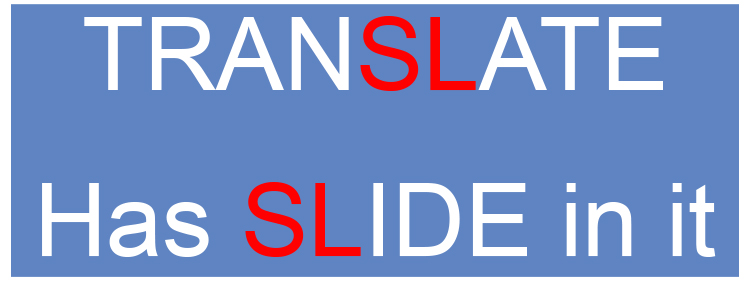And to describe a translation you use a vector like:

Vector ((4),(3))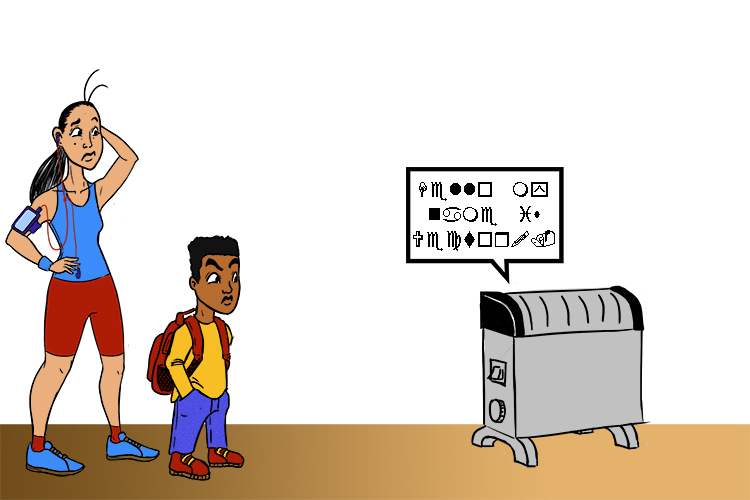Can you translate a single word that heat convector (vector) says?

When we translate an object in maths every vertex (corner) must be moved in the same way.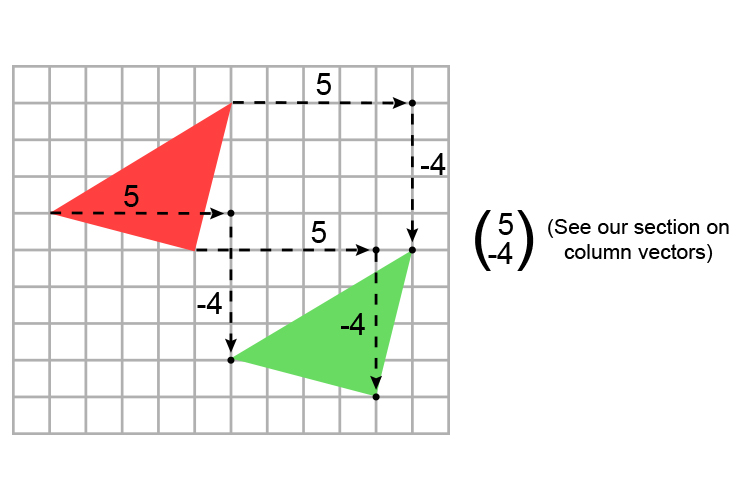This triangle has been translated (slid) 5 along the (xaxis) and -4 down (yaxis).

NOTE:

The object is exactly the same size and shape.

## Labelling the vertices (corners)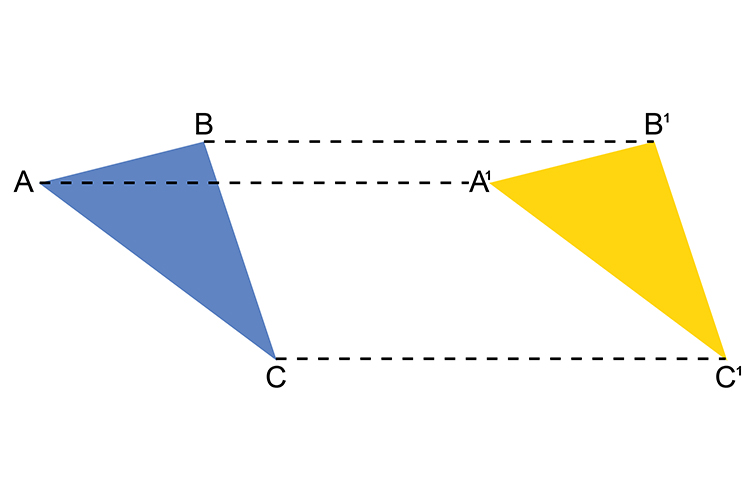The original object in blue has been translated to the right and has been marked yellow. You must note that the new image has been labelled A^1 B^1 \and \ C^1  which is the standard convention.

## Translation Examples

Example 1

Translate triangle A  by the vector ((1),(5))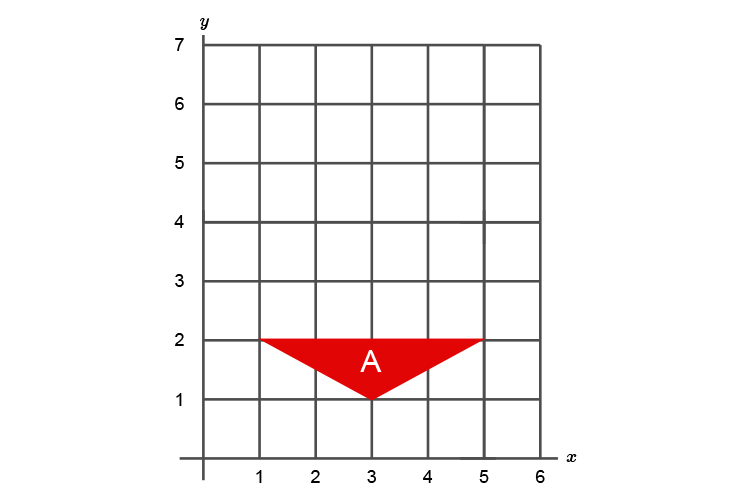Therefore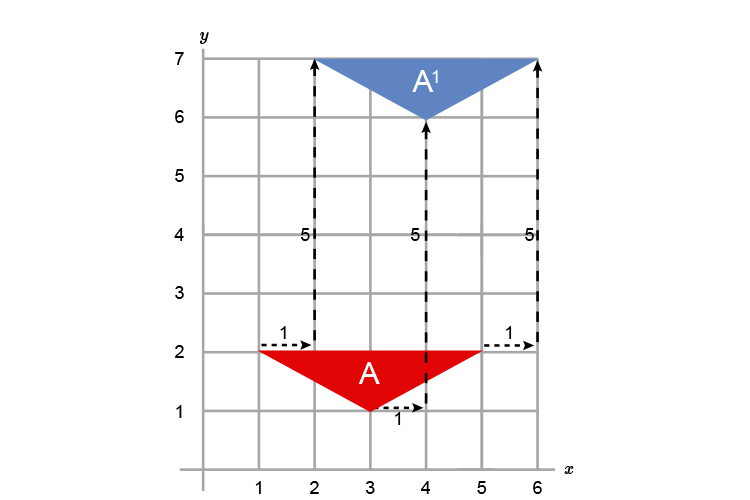Example 2

Translate the following shape by the vector ((-1),(-5))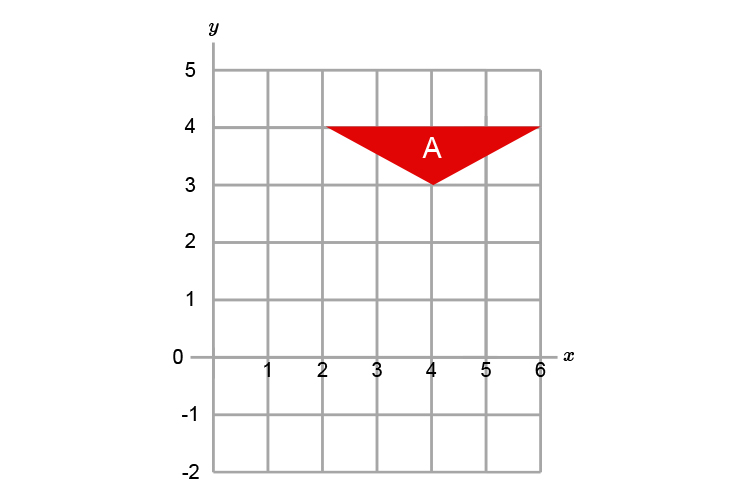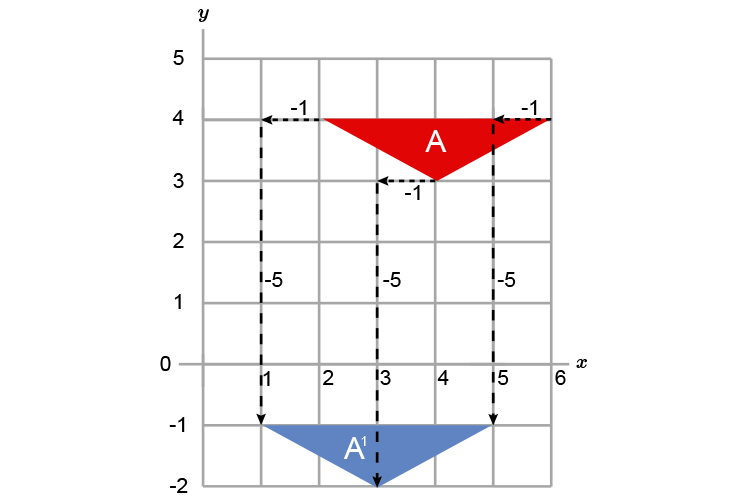Example 3

Translate the following shape by the vector ((-5),(0))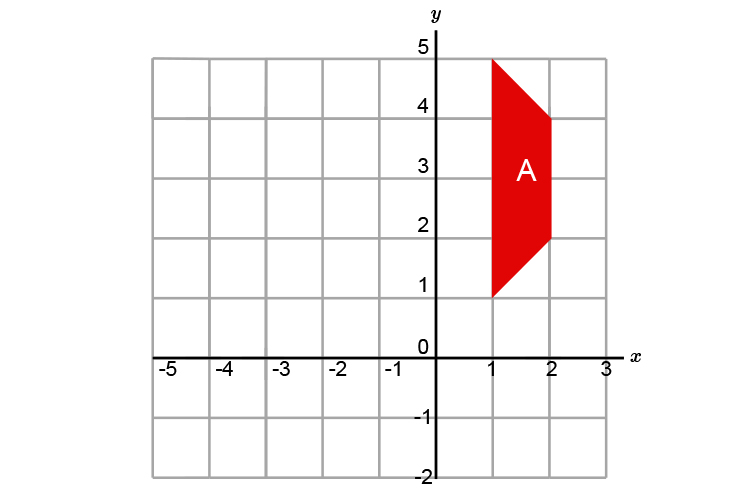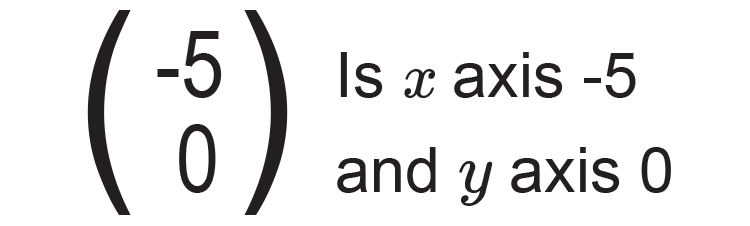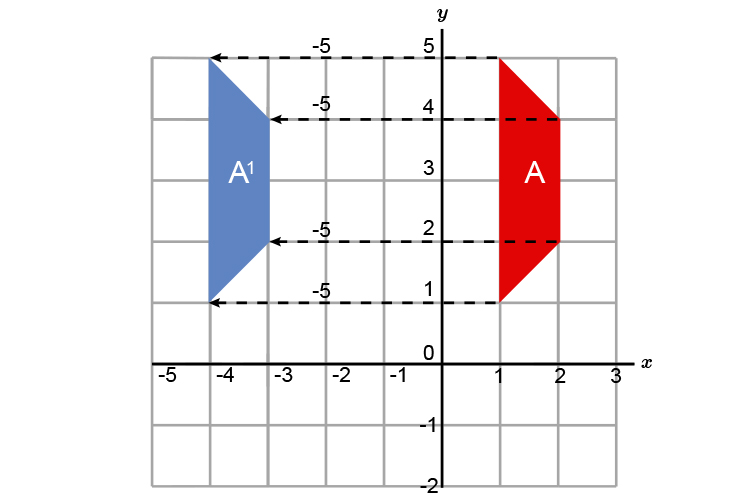Each vertex slides 5 units to the left.

Example 4

What is the displacement vector for the following diagram?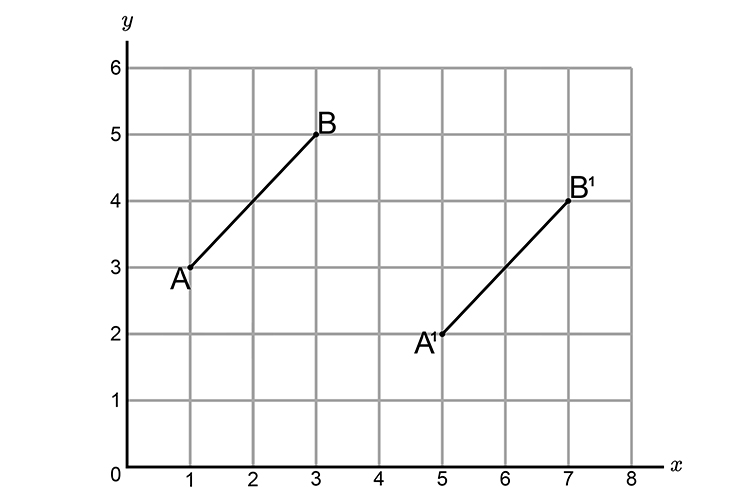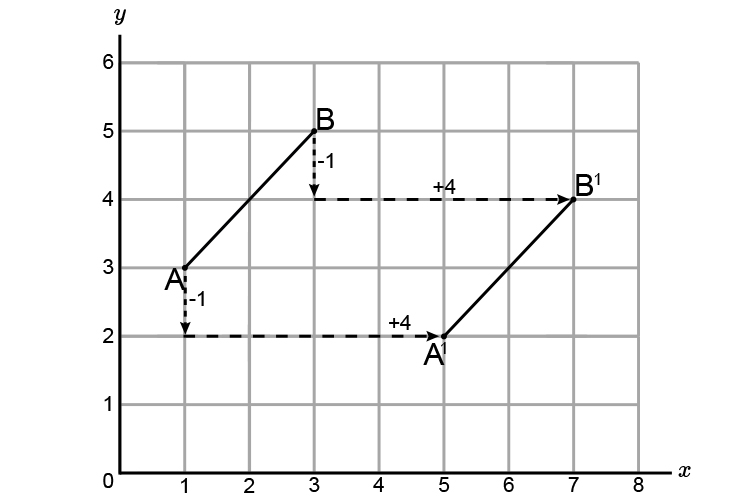The line A\ \ B  has been slid

x  axis +4

y  axis -1

Therefore the displacement vector =((4),(-1))

Example 5

Describe the single transformation that maps A\ to\ B.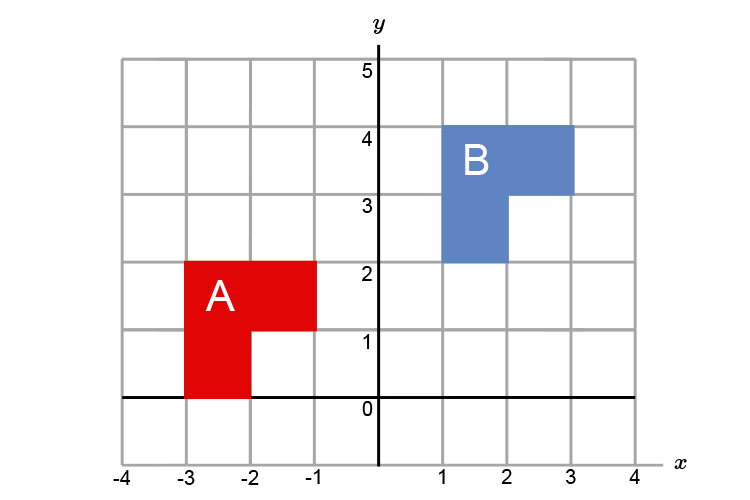You must first describe what this is, and it is a TRANSLATION.

You must now find the displacement vector.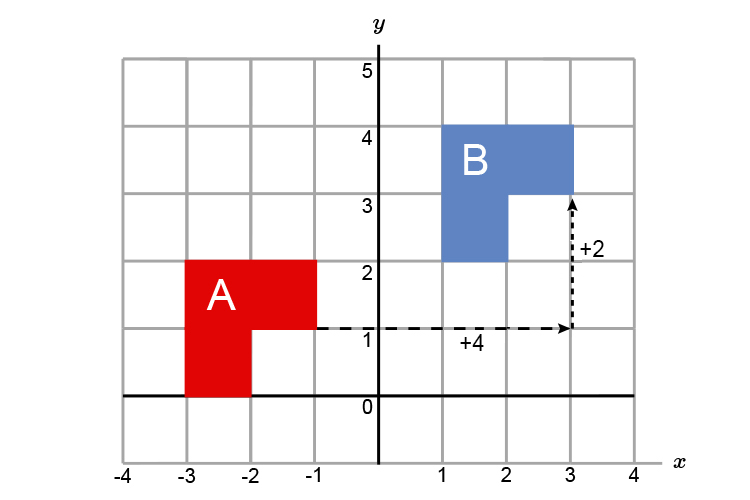The shape has slid,

x axis +4

y axis +2

Therefore the displacement vector =((4),(2))

This is a translation with a displacement vector of ((4),(2))